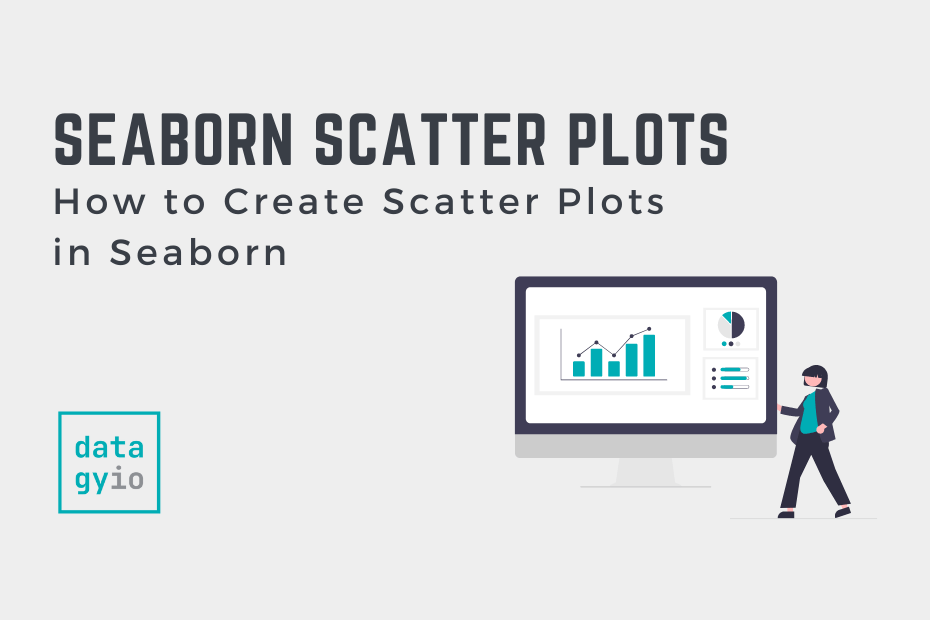# Data Visualization## Seaborn Scatter Plots in Python: Complete Guide

In this complete guide to using Seaborn to create scatter plots in Python, you’ll learn all you need to know to create scatterplots in Seaborn! Scatterplots are an essential type of data visualization for exploring your data. Being able to… Read More »Seaborn Scatter Plots in Python: Complete Guide## How to Create 3D Scatterplots in Matplotlib

In this tutorial, you’ll learn how to make 3D scatterplots using Matplotlib. Being able to visualize data in three dimensions can be an important step in clearly articulating data, especially in science and machine learning. By the end of this… Read More »How to Create 3D Scatterplots in Matplotlib## How to Change Font Size in Matplotlib Plot

In this tutorial, you’ll learn how to change font sizes on a Matplotlib plot. You’ll learn everything you need to know to customize your Matplotlib plot to have exactly the font sizes you want. Data visualization is an incredible tool… Read More »How to Change Font Size in Matplotlib Plot## Set Marker Size in Matplotlib Scatterplots

In this tutorial, you’ll learn how to define and set the marker size in Matplotlib scatterplots. Being able to control the size of your Matplotlib scatterplots allows you to add extra information to your scatterplots. This allows you to communicate… Read More »Set Marker Size in Matplotlib Scatterplots## Creating Pair Plots in Seaborn with sns pairplot

In this tutorial, you’ll learn how to create pair plots in Seaborn, using the sns.pairplot() function. These visualizations plot pairwise relationships in a dataset so that each variable in a dataset will be plotted against each other variable in the… Read More »Creating Pair Plots in Seaborn with sns pairplot## How to Change Plot and Figure Size in Matplotlib

In this tutorial, you’ll learn how to change the plot and figure sizes in Matplotlib. Data visualization is a valuable tool to help you communicate your data. Being able to customize exactly how your plots are sized gives you the… Read More »How to Change Plot and Figure Size in Matplotlib## How to Add Titles to Matplotlib: Title, Subtitle, Axis Titles

In this tutorial, you’ll learn how to add titles to your Matplotlib plots. You’ll learn how to add a title, a subtitle, and axis labels to your plot and subplots. You’ll learn how to style these titles individually and to… Read More »How to Add Titles to Matplotlib: Title, Subtitle, Axis Titles## Pandas Scatter Plot: How to Make a Scatter Plot in Pandas

In this tutorial, you’ll learn how to use Pandas to make a scatter plot. Under the hood, Pandas uses Matplotlib, which can make customizing your plot a familiar experience. Pandas allows you to customize your scatter plot by changing colors,… Read More »Pandas Scatter Plot: How to Make a Scatter Plot in Pandas## Plotting in Python with Matplotlib

In this tutorial, you’ll learn how to get started with plotting in Python with the matplotlib library. You’ll learn how the matplotlib library works and gain an understanding of its “anatomy”. You’ll learn how to plot and customize some simple… Read More »Plotting in Python with Matplotlib## Calculate and Plot a Correlation Matrix in Python and Pandas

In this tutorial, you’ll learn how to calculate a correlation matrix in Python and how to plot it as a heat map. You’ll learn what a correlation matrix is and how to interpret it, as well as a short review… Read More »Calculate and Plot a Correlation Matrix in Python and Pandas## Seaborn Boxplot – How to Create Box and Whisker Plots

Learn how to create a Seaborn boxplot, including how to add styles, titles, axis labels and add grouped boxplots.## Seaborn lineplot – Create Line Plots with Seaborn

Learn how to use the Seaborn line plot andrelplot functions to create beautiful line charts, add titles, customize styles, multiple line charts.